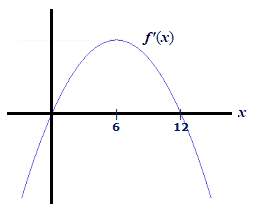# A function f is defined for all x. Use the graph of f' to decide: (a) Over what intervals is f...

## Question:A function {eq}f {/eq} is defined for all {eq}x {/eq}. Use the graph of {eq}f' {/eq} to decide:

(a) Over what intervals is {eq}f {/eq} increasing? Decreasing?

(b) Does {eq}f {/eq} have local maxima or minima? If so, which and where?

## Local Extrema and Intervals of Increase or Decrease:

The sign of the first derivative of a function is instrumental in deciding where the function is increasing or decreasing. The points at which the first derivative is negative (positive) are where the function is decreasing (increasing).

For a differentiable function, its local extrema can occur only at the points

{eq}\bullet {/eq} where the function's first derivative equals zero, and

{eq}\bullet {/eq} around which the sign of the first derivative changes from negative to positive or from positive to negative

## Answer and Explanation:

(a) From the graph since

{eq}\bullet {/eq} the first derivative {eq}f'(x) {/eq} of {eq}f(x) {/eq} is positive on the interval {eq}(0,\,12),\,\, \color{Blue}{f \text{ is increasing on the interval }(0,\,12)\hspace{0.8cm}\mathrm{(Answer)}} {/eq}

{eq}\bullet\,\, f'(x) {/eq} is negative on {eq}(-\infty,\,0)\cup(12,\,\infty),\,\color{Blue}{f \text{ is decreasing on the intervals }(-\infty,\,0)\cup(12,\,\infty)\hspace{2.0cm}\mathrm{(Answer)}} {/eq}

(b) The local extrema of the function {eq}f {/eq} can occur only at its critical points which are those at which the first derivative {eq}f' {/eq} either does not exist or equals zero. Since it is given that the function {eq}f {/eq} is defined everywhere and from the graph {eq}f' {/eq} appears to be a parabola that opens below, we conclude that the first derivative {eq}f' {/eq} of {eq}f {/eq} exists for all real numbers. Hence, the only critical points of {eq}f {/eq} are where

{eq}\begin{align*} f'(x)&=0\\[2ex] \Rightarrow x&=0,\,12 \end{align*} {/eq}

From the graph it is clear that the first derivative of the function {eq}f {/eq} changes

from positive to negative at {eq}\displaystyle \color{Blue}{12\hspace{0.8cm}\mathrm{(point\,\,of\,\,local\,\, maximum)}} {/eq}

and

from negative to positive at {eq}\displaystyle \color{Blue}{0\hspace{0.8cm}\mathrm{(point\,\,of\,\,local\,\, minimum)}} {/eq}